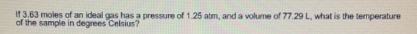# Problem: If 3.63 moles of an ideal gas has a pressure of 1.25 atm, and a volume of 77.29 L. What is the temperature of the sample in degrees Celsius?

###### FREE Expert Solution

Using the ideal gas law: PV=nRT

98% (285 ratings)###### Problem Details

If 3.63 moles of an ideal gas has a pressure of 1.25 atm, and a volume of 77.29 L. What is the temperature of the sample in degrees Celsius?